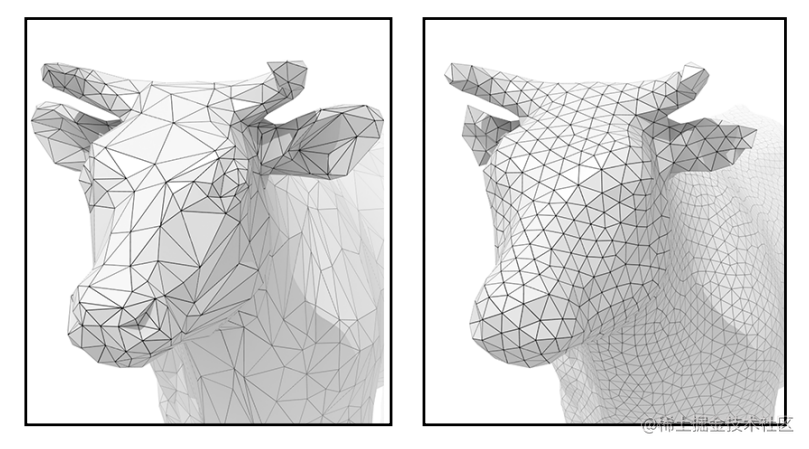GAMES101 - 几何 Geometry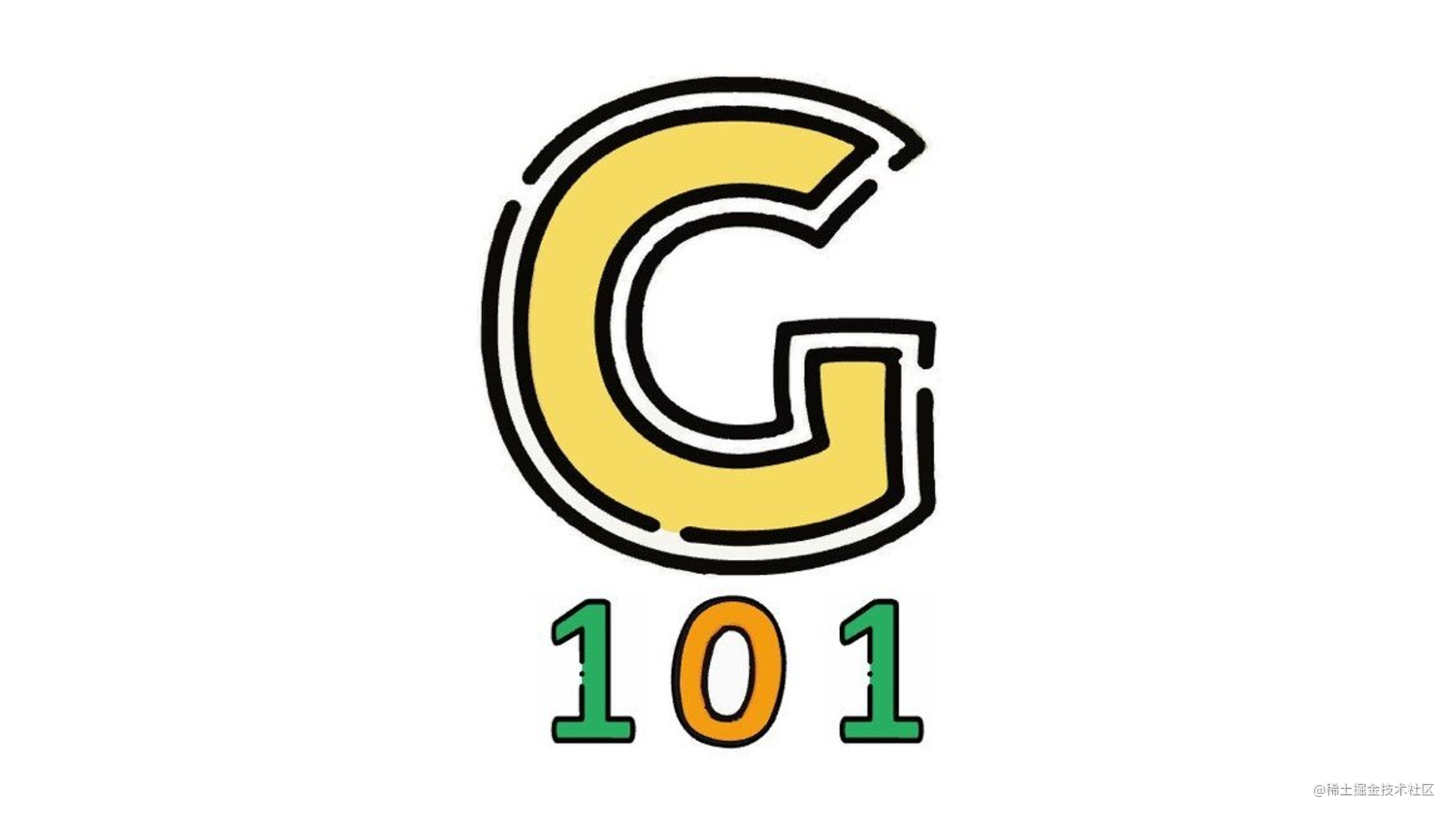隐式几何

$\begin{array}{r} \left(x^{2}+\frac{9 y^{2}}{4}+z^{2}-1\right)^{3}= x^{2} z^{3}+\frac{9 y^{2} z^{3}}{80} \end{array}$构造实体几何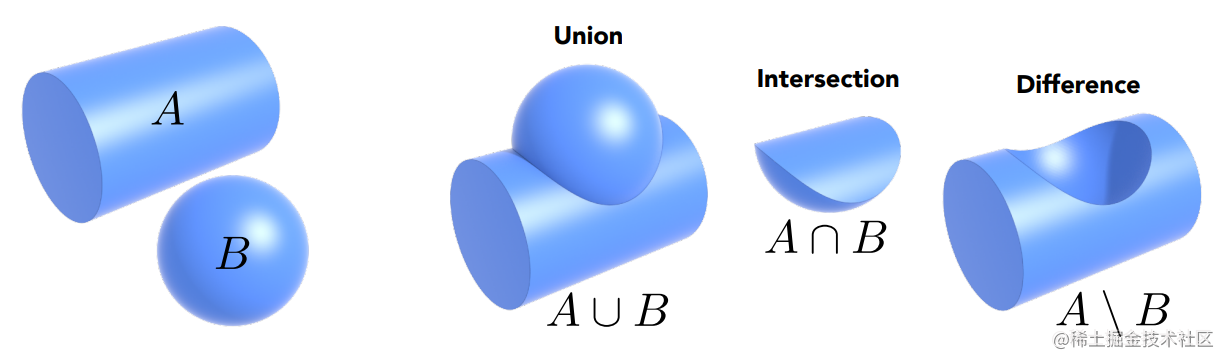距离函数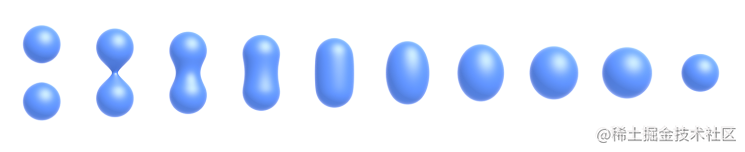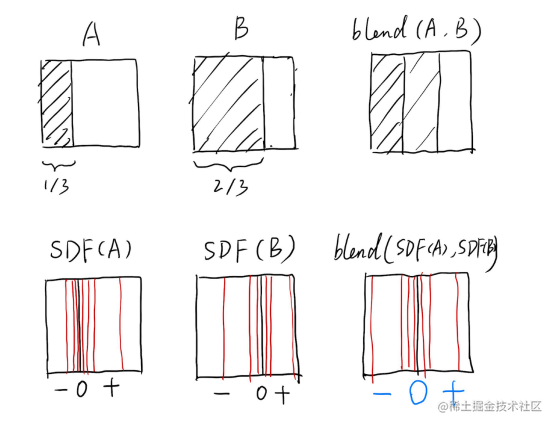水平集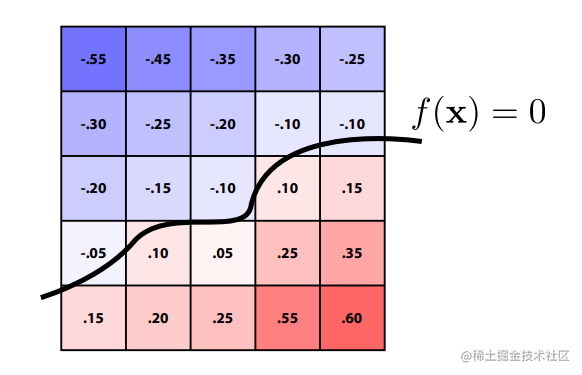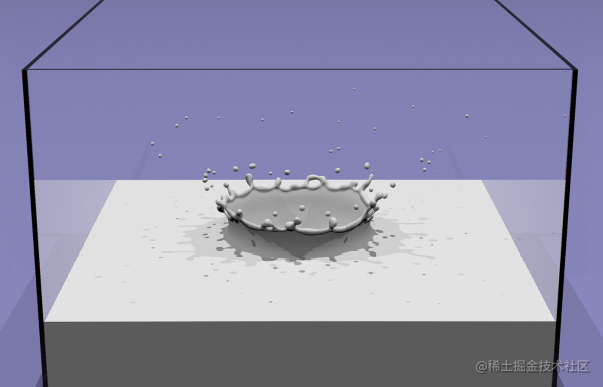分形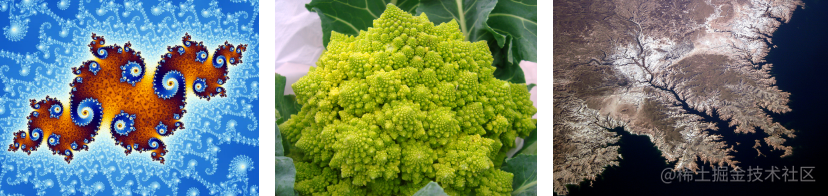显式几何

参数映射

$f: \mathbb{R}^{2} \rightarrow \mathbb{R}^{3} ;(u, v) \mapsto(x, y, z)$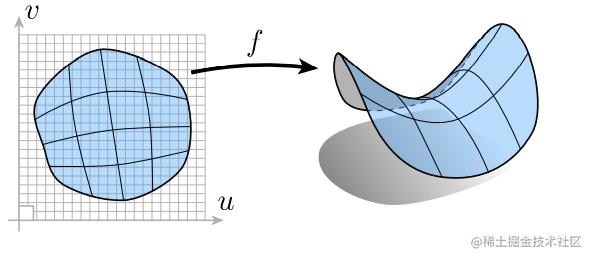多边形面

OBJ 文件把空间中的顶点坐标、法向量和纹理坐标分开表示，再组合起来。对于自动建模导出的 OBJ 文件中实际上会有许多重复的、冗余的数据。OBJ 文件 通过使用 f(face)顶点/纹理坐标/法线 的形式来组合出三角形面的三个顶点，每三个顶点组成一个面。很显然，相邻的面往往会共用顶点，面与面直接就组合了起来形成模型。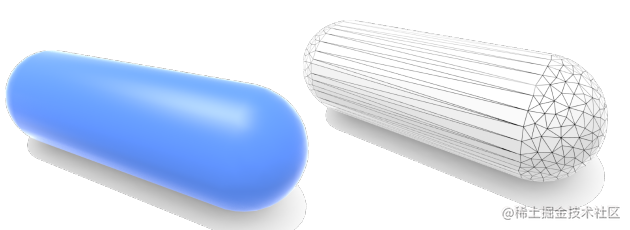点云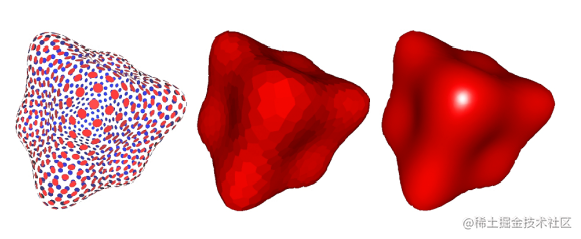贝塞尔曲线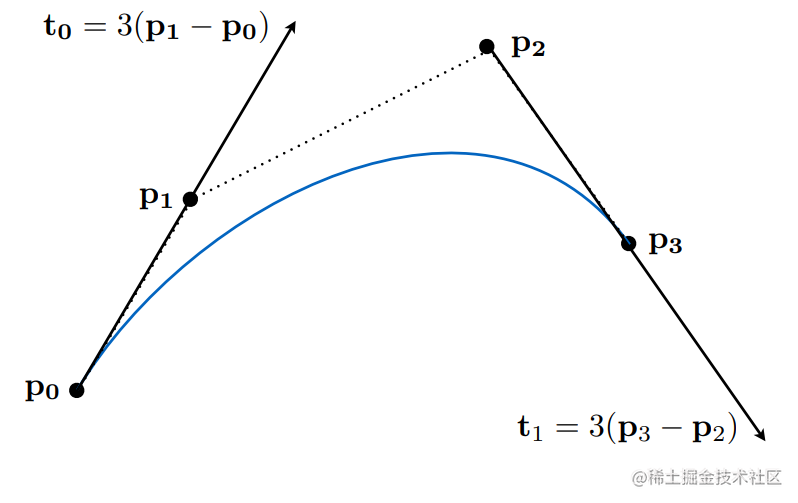德卡斯特里奥算法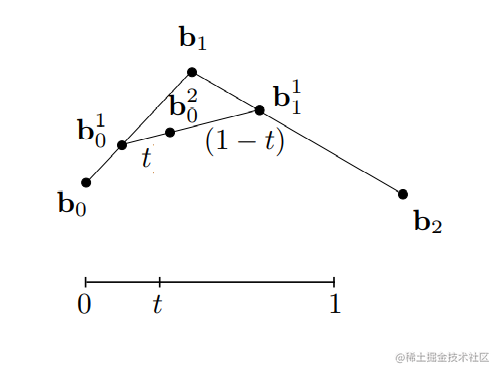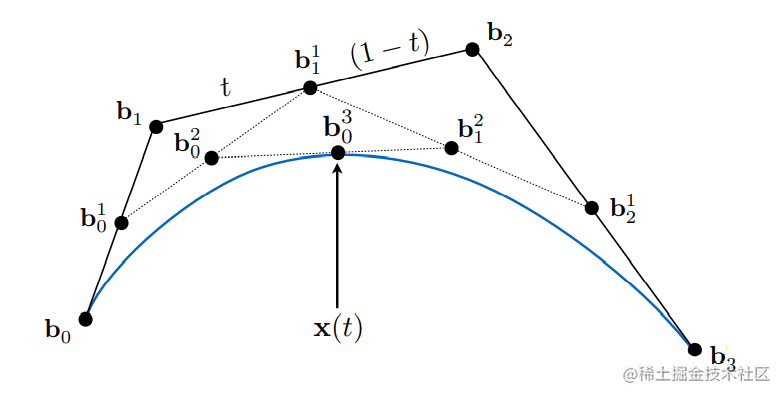贝塞尔曲线的代数表示

$\begin{array}{l}\mathbf{b}_{0}^{1}(t)=(1-t) \mathbf{b}_{0}+t\mathbf{b}_{1} \end{array}$
$\begin{array}{l} \mathbf{b}_{1}^{1}(t)=(1-t) \mathbf{b}_{1}+t \mathbf{b}_{2} \end{array}$
$\begin{array}{l} \mathbf{b}_{0}^{2}(t)=(1-t) \mathbf{b}_{0}^{1}+t \mathbf{b}_{1}^{1} \end{array}$
$\mathbf{b}_{0}^{2}(t)=(1-t)^{2} \mathbf{b}_{0}+2 t(1-t) \mathbf{b}_{1}+t^{2} \mathbf{b}_{2}$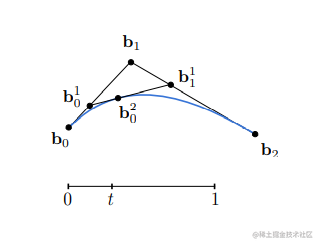$\mathbf{b}^{n}(t)=\mathbf{b}_{0}^{n}(t)=\sum_{j=0}^{n} \mathbf{b}_{j} B_{j}^{n}(t)$
$B_{i}^{n}(t)=\left(\begin{array}{c} n \\ i \end{array}\right) t^{i}(1-t)^{n-i}$

三维坐标中的代数表示

$\mathbf{b}^{n}(t)=\mathbf{b}_{0}(1-t)^{3}+\mathbf{b}_{1} 3 t(1-t)^{2}+\mathbf{b}_{2} 3 t^{2}(1-t)+\mathbf{b}_{3} t^{3}$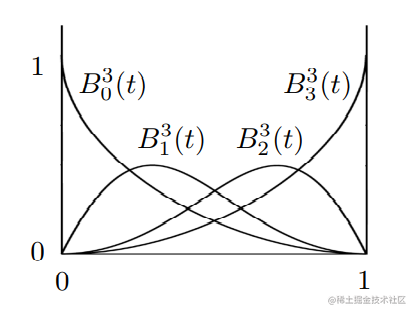贝塞尔曲线的性质

1. 贝塞尔曲线必须过起点和终点。
$\mathbf{b}(0)=\mathbf{b}_{0} ; \quad \mathbf{b}(1)=\mathbf{b}_{3}$
1. 三阶贝塞尔曲线的起始位置的切线和结束位置的切线一定是：
$\mathbf{b}^{\prime}(0)=3\left(\mathbf{b}_{1}-\mathbf{b}_{0}\right)$
$\mathbf{b}^{\prime}(1)=3\left(\mathbf{b}_{3}-\mathbf{b}_{2}\right)$
1. 直接对不同的顶点做仿射变换，重新对变换后的顶点画贝塞尔曲线，一定和通过原始的控制点画出的贝塞尔曲线是一模一样的。也就是说对一个贝塞尔曲线进行仿射变换只需要对其控制点进行仿射变换即可，而不用对所有的点进行变换。相反的是，对贝塞尔曲线做投影变换是会导致贝塞尔曲线发生变换的。

2. 凸包性质：任何一个贝塞尔曲线，任何一个点，在任意时间 t 一定都在给定的几个控制点所形成的凸包 (Convex Hull) 里。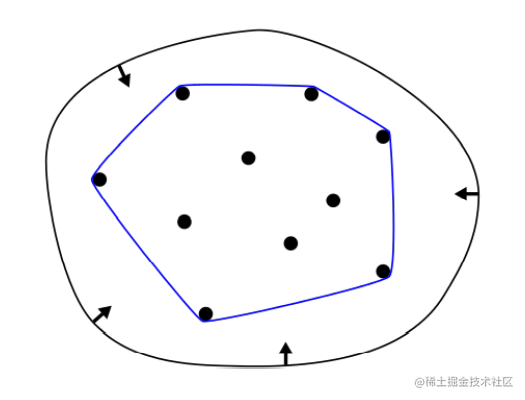分段贝塞尔曲线

连续性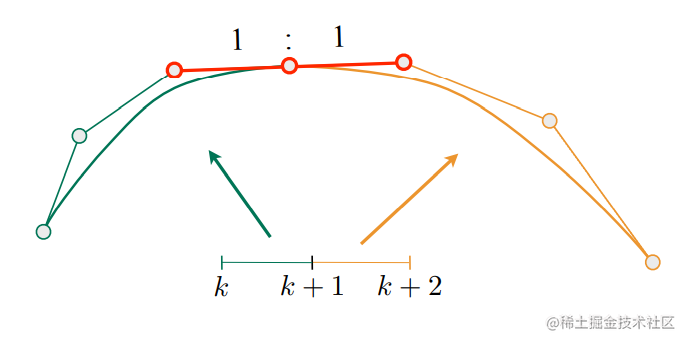贝塞尔曲面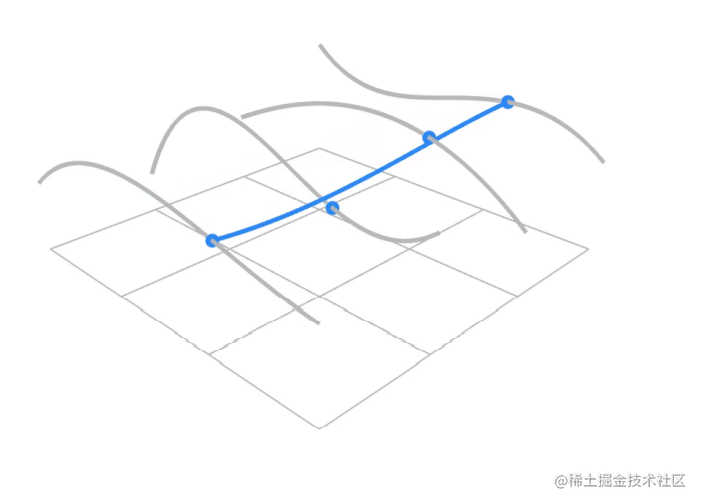贝塞尔曲面坐标评估

1. 使用 de Casteljau 算法 评估点在时间 $u$ 时，在四条贝塞尔曲线上的位置，即可得到新的四个控制点。

2. 然后再使用一次 de Casteljau 算法 ，评估出点在时间 $v$ 时 所形成的贝塞尔曲线。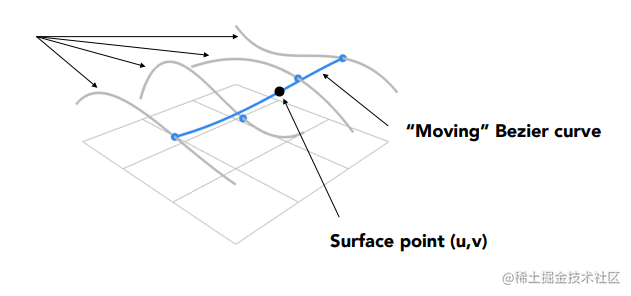网格细分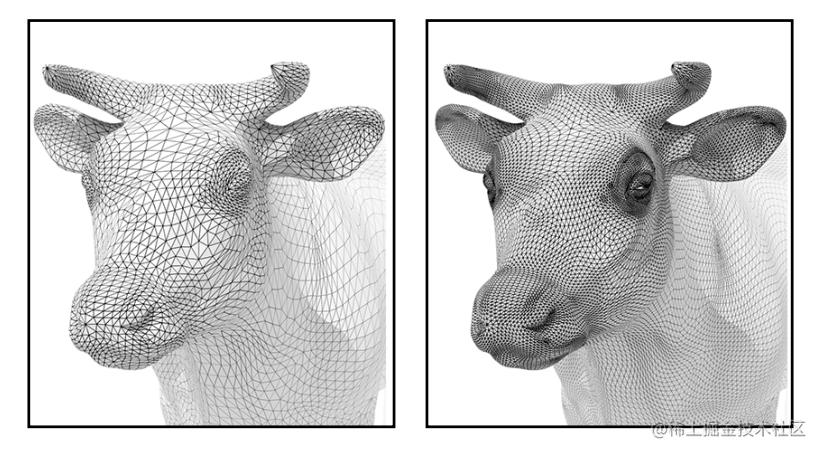卢氏细分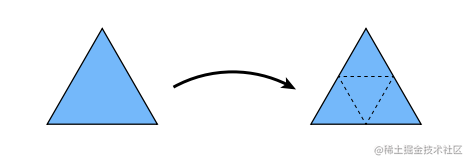新顶点的更新

$P = 3 / 8^{*}(A+B)+1 / 8^{\star}(C+D)$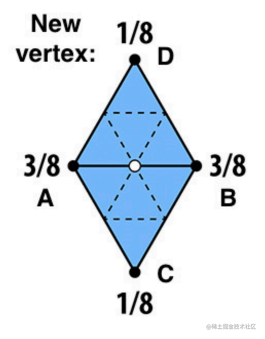旧顶点的更新

$(1 - n · u ) · P_{o} +\mathrm{u} · P_{sum(n)}$

Catmull-Clark 细分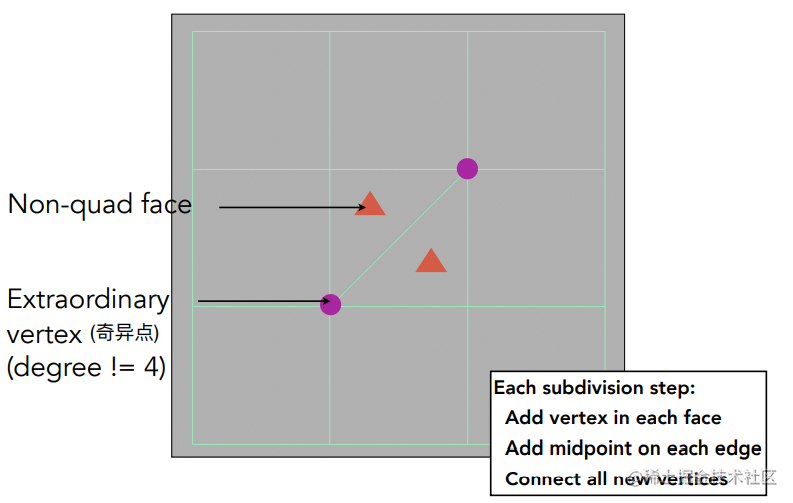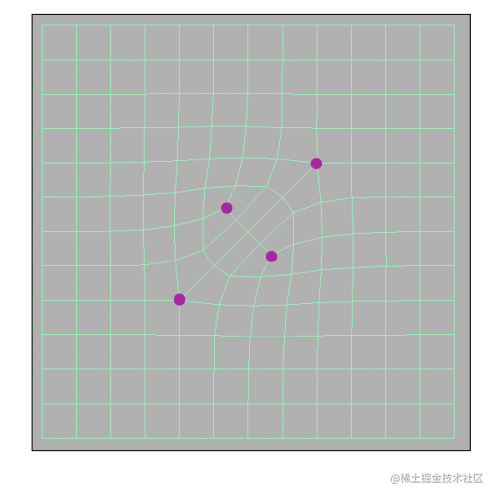顶点的更新

Loop 细分 有所不同的是 Catmull-Clark 细分 将顶点分为三类进行更新。

• 在面中心的点 (Face Point)
• 在边中心的点 (Edge Point)
• 旧顶点 (Old Vertex Point)

Catmull-Clark 细分 并按顺序对三类顶点进行加权平均计算，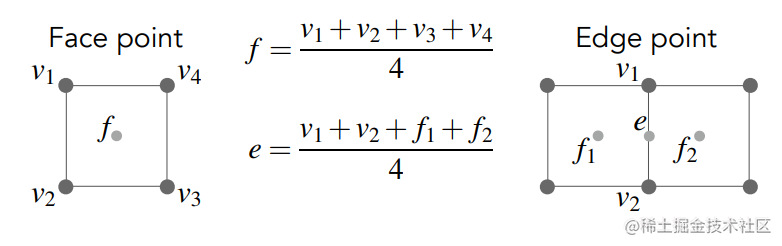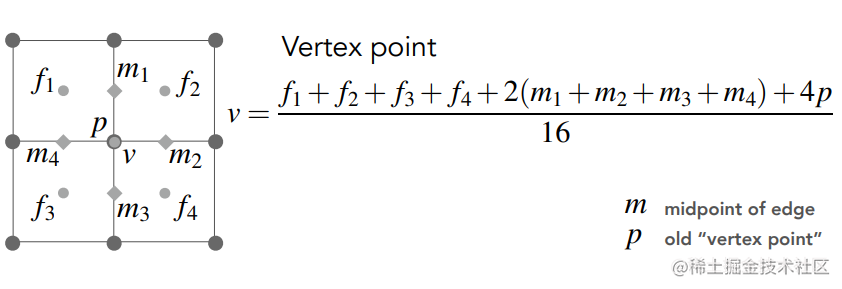网格简化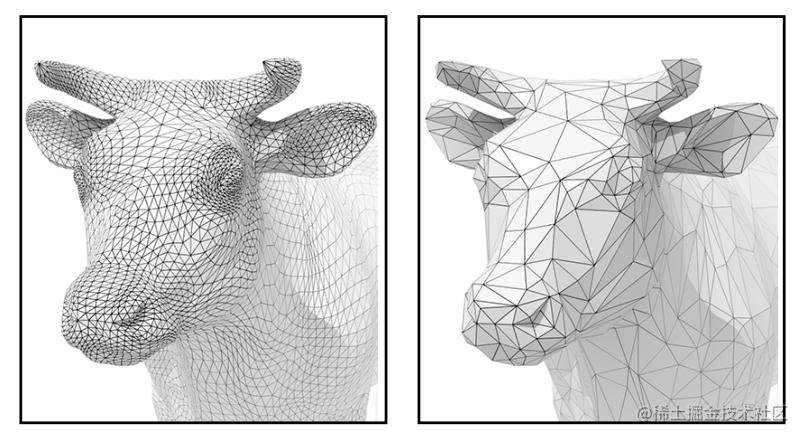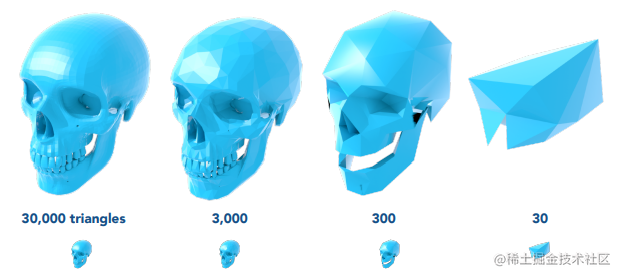边坍缩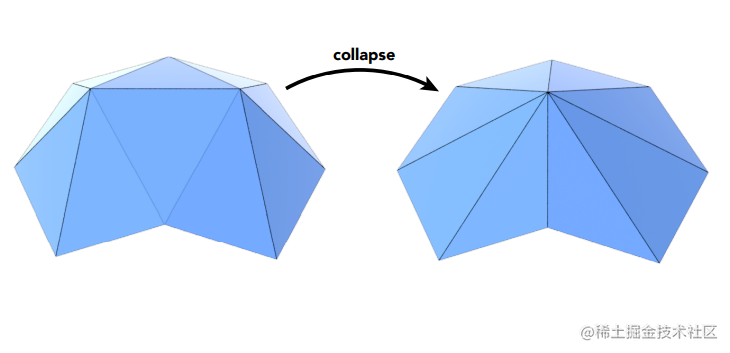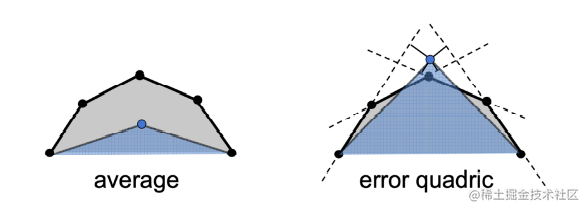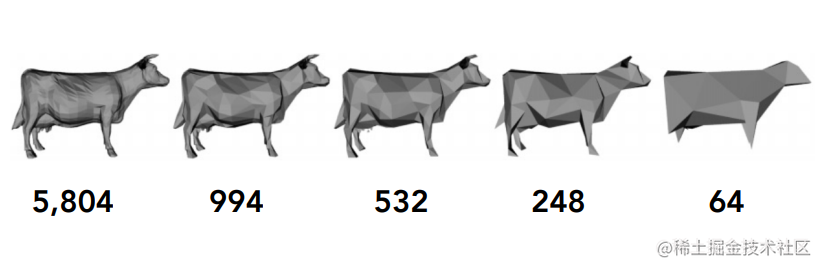网格规范化